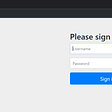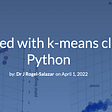# Kolmogorov Smirnov Two Sample Test with Python

Statistics offers a plethora of tests which are frequently used by students, academicians and industry participants for various purposes including testing for independence, goodness of fit, etc. In the recent times with the explosion of data, we are presented with volumes of data that was never thought of before. Statistical tests have also evolved over the years thereby enabling them to be applied to numerous problems encountered in business applications. One such test which is popularly used is the Kolmogorov Smirnov Two Sample Test (herein also referred to as “KS-2”). In the first part of this post, we will discuss the idea behind KS-2 test and subsequently we will see the code for implementing the same in Python. Basic knowledge of statistics and Python coding is enough for understanding this post.

Consider we are presented with two sets of data. We wish to run a statistical test to check that two data samples come from the same distribution. In such a scenario Kolmogorov Smirnov Sample Test comes to our help. The advantage of this test is that it’s non-parametric in nature, therefore it is distribution agnostic. Thus, we are not concerned if the underlying data follows any specific distribution. Further, there are two versions of the Kolmogorov Smirnov Sample Test that are available and we need to choose the one that suits our requirement. Although we will be implementing the KS-2 Test in Python in this post, it also makes sense to talk about KS-1 Test so that we are familiar with the conceptual differences between the two tests.

· One Sample Kolmogorov Smirnov Test (KS-1):

This test is based on empirical distribution function. So, if we are given N data points k1, k2, k3……kn. When we plot the data points, the graph is like a step function. We then superimpose this empirical distribution function against a cumulative distribution function for a given distribution. The conclusions that we derive from these graphs is based on the maximum distance between the two distribution function graphs.

· Two Sample Kolmogorov Smirnov Test (KS-2):

Under KS-2 test, we do not compare an empirical distribution function against a cumulative distribution function. Rather, we assume two empirical distributions and then take a difference between them. Thus, the beauty of KS-2 Test lies in the fact that we do not need to know / assume any specific distribution. We proceed with calculating the difference between the two empirical distributions at each of the data points from our data set.

Mathematically, D = |E1(k)-E2(k)|, where

D = absolute value of the distance between two data sets

E1(k)-E2(k): E1(k) and E2(k) are computed at each point in the given data set.

We define the hypothesis test as under:

i) H0 (null hypothesis): Two samples are from the same distribution

ii) Ha (alternative hypothesis): Two samples come from different distributions

iii) The Test statistic that we will test is D = |E1(k)-E2(k)|

iv) Level of significance (alpha): A critical value table for KS-2 Test is used for comparing the test statistic D against the critical value for a given level of significance from the table Alpha is generally assumed to be 0.05.

Now we will implement the KS-2 Test in Python by using a hypothetical data set.

The hypothetical dataset is given below:

Data 1 | Data 2 | |
10353 | 10427 | |
10874 | 10147 | |
10777 | 10689 | |
10956 | 10141 | |
10975 | 10247 | |
10335 | 10986 | |
10191 | 10163 | |
10937 | 10684 | |
10017 | 10300 | |
10995 | 10032 | |
10265 | 10762 | |
10730 | 10365 | |
10511 | 10516 | |
10492 | 10695 | |
-11984 | -11351 | |
-11903 | -11198 | |
-11556 | -11492 | |
-11052 | -11892 | |
-11428 | -11353 | |
-11155 | -11977 | |
-11899 | -11026 | |
-11686 | -11719 | |
-11737 | -11866 | |
-11111 | -11310 | |
-11290 | -11060 | |
-11469 | -11579 | |
-11492 | -11185 | |
-11464 | -11583 | |
-11006 | -11765 | |
-11555 | -11953 | |

Python code for the same is given below:

We leverage the Scipy library from Python. Scipy has the KS-2 model implemented, we need to call this method and use it in our program.

You may create your data sets in the above format and save it in a .csv file and name the same as DataSet.csv . Subsequently you may run the below code to get the output.

import pandas as pd
from scipy.stats import ks_2samp

data1 = df.iloc[:,0]
data2 = df.iloc[:,1]
test = ks_2samp(data1,data2)
print(test)

Once we run the above piece of code, we get the output of the KS-2 Test which includes: The value of the test statistic and the p-value. Below is our output.

Ks_2sampResult(statistic=0.16666666666666663, pvalue=0.7600465102607566)

Our job now is to compare the statistic value against the critical value arrived at by using the table provided in this link: Critical value table for KS-2 test

For our sample: n1= 30, n2=30 , alpha = 0.05

Thus, using the formula given in the document, the critical value for D(alpha) = 1.36 * 0.2581 = 0.3510

Next, we compare our test statistic (0.1666) given above against the critical value D(alpha) (0.3510) computed above.

The result is interpreted as:

i) If statistic > critical value: then two samples come from different distributions

ii) If statistic < critical value: then two samples come from same distributions

We observe that in our example, the two samples come from same distribution.

--

--

--

## More from Ameya Abhyankar

Founder: FinQuest Institute | Ekspert Consulting; www.finquestinstitute.com; www.ekspertconsulting.com

Love podcasts or audiobooks? Learn on the go with our new app.

## Introducing ODP.NET 21c: EF Core 5, JSON, and More## Python Text Analysis With the Schrutepy Package## Linux file system## Fibery.io Private Beta Release is here## The March “powering up” dev update## Spring Security Series — Securing Spring Boot Todo Application (Part 2)## Ameya Abhyankar

Founder: FinQuest Institute | Ekspert Consulting; www.finquestinstitute.com; www.ekspertconsulting.com

## Getting to know PyScript## Getting started with k-means clustering in Python## How to Detect-le-Defect on Twitch